## Summary and examples

A standard quadratic inequality has the same form as the corresponding equation with an inequality sign instead of an equal sign, see the following example: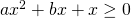Of course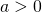, because otherwise it is no quadratic inequality.

In Quadratic equations (factorizing) and Quadratic equations (abc-formula) we discussed extensively how this type of equations could be solved. It is advised to study these topics first before you continue with quadratic inequalities.

When solving quadratic inequalities, we must always make use of solving quadratic equations. Both methods, factorization or applying the abc-formula, can be used.

The approach is as follows. We calculate the solutions of the corresponding equation, and the value of the discriminant: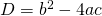is important.

Next, we make use of the fact whether the graph of the quadratic function is an 'opens up' or an 'opens down' parabola.

We distinguish three cases:

1.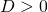In this case the solutions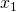and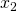are different. The parabola intersects the-axis in two different points. Then, depending on('opens up' or 'opens down') we know for which values ofthe function is greater or less than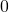is.

2.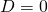In this case the solutionsandare equal. For this value ofthe function equals, for other values ofit is greater than('opens up') or less than('opens down').

3.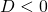In this case the equation has no (real) solutions. The parabola lies entirely above ('opens up') or below ('opens down') the-axis.

##### Example 1

Solve: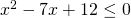We immediately see that the left-hand side can be factorized: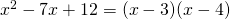and the equation: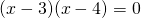has the following solutions: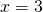or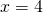The parabola 'opens up', has two intersection points with the-axis and thus the inequality is satisfied for: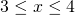We would have found the same result when applying the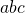-formula.

##### Example 2

Solve: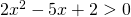In this case we find the solutions of the equation: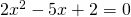by applying the-formula: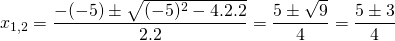so: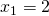or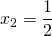The graph of the function in the left-hand side is an 'opens up' parabola and thus the inequality is satisfied by the following values of:or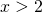##### Example 3

Solve: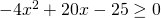First we solve the equation: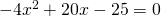or: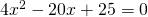Applying the-formula we get: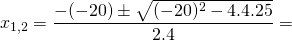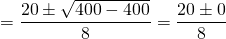Thus the solution is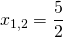. The equation has two coinciding solutions, sometimes it is said that the equation has only one solution.
The graph of the function in the left-hand side is an 'opens down' parabola and thus the inequality is only satisfied for: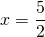Note that this solution could also be found by applying the special product:##### Example 4

Solve: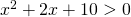First we solve the corresponding equation: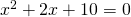The discriminant has the value: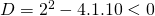Thus the equation has no solutions and sincethe graph of the function in the left-hand side lies entirely above the-axis. So the inequality is satisfied for all values of.

##### Example 5

Solve: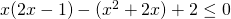We rewrite this equation: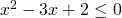and solve the corresponding equation: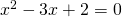Factorization yields: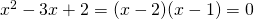and thus we have the following solutions: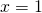or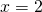The graph is an 'opens up' parabola and thus the inequality is satisfied for the following values of: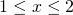0